# How to implement insertion sort in JavaScript?

## Insertion Sort

The Insertion Sort is a sorting algorithm that works very similar to the way we sort the playing cards when we play. The arrangement of elements in a sorted manner. In this algorithm, the array will be divide virtually into two parts which are sorted and unsorted parts. The values present in unsorted part will be pick and placed at the correct position where it satisfies the sorting order.

The Insertion sort is simple algorithm having simple implementation. Generally, this algorithm is efficient for smart data values. This is suitable for data sets which are already partly sorted.

Let’s see some input and output scenarios

Consider an array having some elements in it in a random order which are not sorted, we can sort the elements by performing insertion sort. So, Let’s check the scenario below.

Input = [24, 22, 26, 10, 12];
Output = 10, 12, 22, 24, 26


## How insertion sort algorithm works?

To know how the merge sort is working, let’s assume an array arr=[24, 22, 26, 10, 12].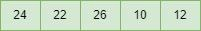### First Pass

• At first in insertion sort, the initial two elements in the array are compared first.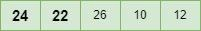• 22 is greater than 24 here, thus they are not in ascending order and 24 is not in a correct position. So swap 22 and 24. And for now 24 is stored in a sub array.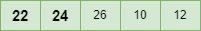### Second Pass

• Now, compare the next two elements in the array.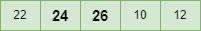• Here, both the elements 24 and 26 are in ascending order as 26 is greater than 24. Thus no swapping will be occurred.

• 24 is also stored in the sub array along with 22.

### Third Pass

• Present there are two elements 22 and 24 are in sub-array.

• Now compare the next two elements 10 and 26.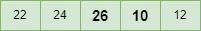• As 10 is smaller than 26, Swap both the values.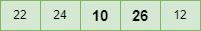• Even after swapping 10 and 24 are sorted, thus swap again.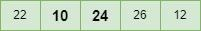• Again 10 and 22 are not sorted, so swap again.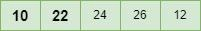• Now, 10 is at correct position.

### Fourth Pass

• Currently, the elements in the sorted sub array are 10, 22 and 24.

• Comparing the next two elements 26 and 12.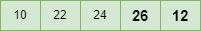• As they are not sorted, swap both the values.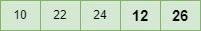• Now, 12 is smaller than 24. Thus swap them.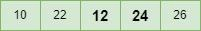• Here 12 is smaller than 22 and they are not sorted, so swap them.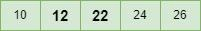• Done, the array is perfectly sorted.

### Algorithm

To sort an array sized n in ascending order using insertion sort algorithm.

• If it is the first element, it is already sorted. return 1;

• Pick next element

• Compare with all elements in the sorted sub-list

• Shift all the elements in the sorted sub-list that is greater than the value to be sorted

• Insert the value

• Repeat until list is sorted

### Example 1

Following is an example of insertion sort −

<!DOCTYPE html>
<html>
<title>Insertion Sort in JavaScript</title>
<script>
function insertion_Sort(Array, arr_len) {
for (let x = 1; x < arr_len; x++) {
let item = Array[x];
let y = x - 1;
while (y >= 0 && Array[y] > item) {
Array[y + 1] = Array[y];
y = y - 1;
}
Array[y + 1] = item;
}
}
document.write("The sorted array will be: ");
function SortedArray(Array, arr_len) {
for (let x = 0; x < arr_len; x++)
document.write(Array[x] + " ");
}
let Array = [24, 22, 26, 10, 12];
let arr_len = Array.length;
insertion_Sort(Array, arr_len);
SortedArray(Array, arr_len);
</script>
<body>
</body>
</html>


### Example 2

Using the unshift() method

This method is used to add one more elements to an array at the starting position. It returns the current length of the array.

<html>
<script>
function iSort(array) {
for (var p = 1; p < array.length; p++) {
if (array[p] < array){
array.unshift(array.splice(p,1));
}
else if (array[p] > array[p-1]) {
continue;
}
else {
for (var q = 1; q < p; q++) {
if (array[p] > array[q-1] && array[p] < array[q]){
array.splice(q,0,array.splice(p,1));
}
}
}
}
return array;
}
document.write(iSort([0,-3,5,8,2,7,6]));
</script>